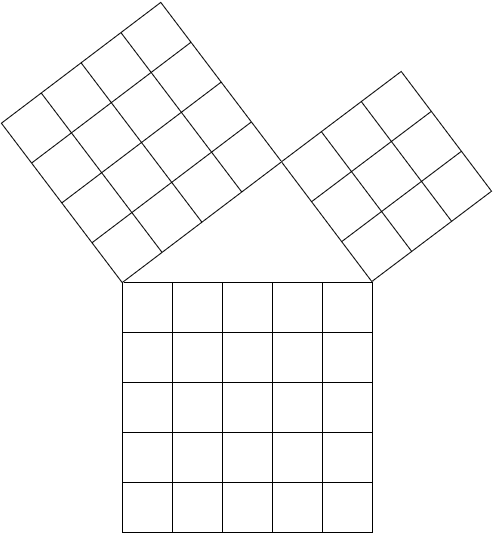# A Famous Exponential$\large 3^x +4^x=5^x$

Find the sum of all possible positive integers $x$ so that the equation is true.

×